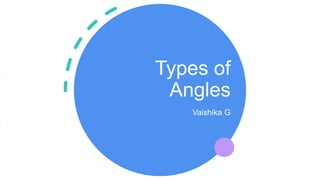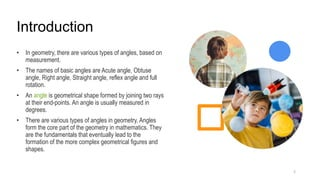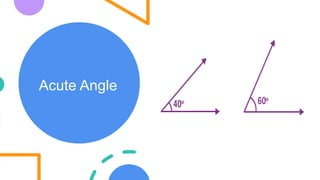Ce diaporama a bien été signalé.
Le téléchargement de votre SlideShare est en cours. ×

# Types of Angles.pptx

Publicité
Publicité
Publicité
Publicité
Publicité
Publicité
Publicité
Publicité
Publicité
Publicité
PublicitéChargement dans…3
×

## Consultez-les par la suite

1 sur 15 Publicité

# Types of Angles.pptx

In geometry, there are various types of angles, based on measurement. The names of basic angles are Acute angle, Obtuse angle, Right angle, Straight angle, reflex angle and full rotation. An angle is geometrical shape formed by joining two rays at their end-points. An angle is usually measured in degrees.

There are various types of angles in geometry. Angles form the core part of the geometry in mathematics. They are the fundamentals that eventually lead to the formation of the more complex geometrical figures and shapes.
What are Angles
When two rays combine with a common endpoint and the angle is formed. The two components of an angle are “sides” and “vertex”.
Parts of Angle
Vertex – Point where the arms meet.
Arms – Two straight line segments form a vertex.
Angle – If a ray is rotated about its endpoint, the measure of its rotation is called angle between its initial and final position.
Classification of Angles
Angles can be classified into two main types:

Based on Magnitude
Based on Rotation
Six Types of Angles
In Maths, there are mainly 5 types of angles based on their direction. These five angle types are the most common ones used in geometry. These are:

Acute Angles
Obtuse Angles
Right Angles
Straight Angles
Reflex Angles
Full Rotation

In geometry, there are various types of angles, based on measurement. The names of basic angles are Acute angle, Obtuse angle, Right angle, Straight angle, reflex angle and full rotation. An angle is geometrical shape formed by joining two rays at their end-points. An angle is usually measured in degrees.

There are various types of angles in geometry. Angles form the core part of the geometry in mathematics. They are the fundamentals that eventually lead to the formation of the more complex geometrical figures and shapes.
What are Angles
When two rays combine with a common endpoint and the angle is formed. The two components of an angle are “sides” and “vertex”.
Parts of Angle
Vertex – Point where the arms meet.
Arms – Two straight line segments form a vertex.
Angle – If a ray is rotated about its endpoint, the measure of its rotation is called angle between its initial and final position.
Classification of Angles
Angles can be classified into two main types:

Based on Magnitude
Based on Rotation
Six Types of Angles
In Maths, there are mainly 5 types of angles based on their direction. These five angle types are the most common ones used in geometry. These are:

Acute Angles
Obtuse Angles
Right Angles
Straight Angles
Reflex Angles
Full Rotation

Publicité
Publicité

### Types of Angles.pptx

1. 1. Types of Angles Vaishika G
2. 2. Introduction 2 • In geometry, there are various types of angles, based on measurement. • The names of basic angles are Acute angle, Obtuse angle, Right angle, Straight angle, reflex angle and full rotation. • An angle is geometrical shape formed by joining two rays at their end-points. An angle is usually measured in degrees. • There are various types of angles in geometry. Angles form the core part of the geometry in mathematics. They are the fundamentals that eventually lead to the formation of the more complex geometrical figures and shapes.
3. 3. Acute Angle
4. 4. Acute angle (contd..) An acute angle is defined as an angle that measures less than 90 degrees i.e. measure between 0° to 90°. Some of the examples are 60°, 30°, 45°, etc. A triangle formed by all the interior angles measuring less than 90° is called an acute triangle. For example, an equilateral triangle is an acute triangle since the interior angles measure 60°. 4
5. 5. Obtuse Angle 5
6. 6. Obtuse angle (contd..) 6 In geometry, an obtuse angle is defined as an angle that is greater than 90° and less than 180°. Often during a day in a 24 hours duration, we can see a clock framing many obtuse angle degrees between a minute hand and an hour hand.
7. 7. Right Angle 7
8. 8. Right angle (contd..) A right angle is an angle that measures 90 degrees. It is the most commonly seen angle in our day-to-day life. It can be seen in the corners of a room, the edges of boxes, the screen of a mobile phone, and so on. The sides of a square and a rectangle always form a right angle with each other. In radians, it is represented as π/2. 8
9. 9. Reflex Angle 9
10. 10. Reflex angle (contd..) 10 A reflex angle is an angle that lies between 180° and 360°. A reflex angle and the corresponding angle that lies on the other side of it together form a complete angle of 360°. It should be noted that a reflex angle always has an acute angle, an obtuse angle, or a right angle on the other side of it.
11. 11. Straight Angle 11
12. 12. Straight angle (contd..) 12 A straight angle measures 180° and looks like a straight line, therefore, it is a mathematical way of expressing a straight line. It is an angle whose arms lie in opposite directions from the vertex and they join together to form 180°. Whenever two rays join together they form an angle and the angle subtended by two rays in opposite directions is called a straight angle.
13. 13. Complete Angle 13
14. 14. Complete angle (contd..) 14 If after one whole rotation, the final ray coincides with the incident or initial ray, then the angle so formed is known as the complete angle. The other names are a full angle and a round angle. The angle is equal to 2π radians = 360 degrees corresponding to the central angle of an entire circle. Four right angles or two straight angles make a complete angle. The angle is similar to a zero angle but the difference is the amount of rotation.
15. 15. Thank you 15 Vaishika G